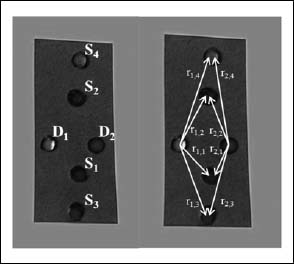Figure 1. A line orientated probe spatial design was used. Left panel: 4 light emitting diodes (S1-4) aligned along the muscle fiber orientation and 2 phase sensitive detectors (D1 and D2). Right panel: source-detector separation distance diagram for each detector (r1,1=1.3 cm, r1,2=1.7 cm, r1,3=2.7 cm, r1,4=3.1 cm, r2,1=1.5 cm, r2,2=1.9 cm, r2,3=2.8 cm, r2,4=3.2 cm).